MACHINES FOR BUILDING SYMMETRY

# MARIA DEDÒ

Maria.Dedo@mat.unimi.it

1.    Introduction

What I am going to describe here is a mathematical interactive exhibition, “Simmetria, giochi di specchi”, based on the theme of symmetry, which has been realized in the last few years by the Mathematics Department of Milan University.

The main objects of this exhibition are six “machines for building symmetry”. Three of them are planar, consisting of three mirrors each, all perpendicular to the plane of the table where they are posed, and cutting on this plane - respectively - an equilateral triangle, a right isosceles triangle, a right triangle with angles p/6 and p/3 (here we shall call them mirror boxes).

The other three are 3-dimensional caleidoscopes; each one again consists of three mirrors, belonging to three planes concurrent in a point O and each one cuts on a sphere with center O a spherical triangle: the first one of these three triangles has angles p/2, p/3 and p/3; the second one has angles p/2, p/3 and p/4, while the third one has angles p/2, p/3 and p/5.Each one of the mirror boxes is provided with some tiles, which are the right ones in order to see in the box a given picture (which will be a tessellation of the plane): some of these pictures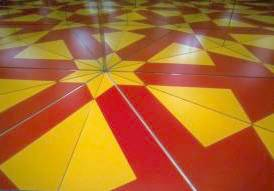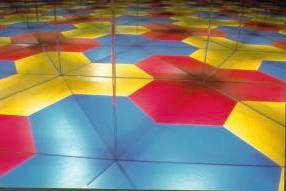are shown on the walls of the box, so that the visitor knows in advance in which one of the three boxes he will be able to build that picture; other ones are shown mixed up in a poster, so that the visitor willing to reconstruct that picture has first of all to decide in which one of the three boxes this can be done. Mathematically, this is a first not trivial problem: this decision corresponds in fact to detecting the symmetry group of the given picture.

The 3-dimensional caleidoscopes can be handled in an analogous way: some small pieces are provided, which correspond to the fundamental domain for the action of the symmetry group on some polyhedra. By putting the piece in the corresponding caleidoscope, the polyhedron is reconstructed.

Both in the 2-dimensional mirror boxes and in the 3-dimensional caleidoscopes the same mathematical concept is underlined: the classification of something (a planar picture in the first case, a solid object in the second one) with respect to its symmetry group. So a “machine for building symmetry” builds up different things, depending on what is put inside it, but all what is built with the same machine has the same kind of symmetry; while different machines build up different symmetries.

The actual exhibition contains in fact other objects, which are all functional to this main idea.

This paper is organized as follows: section 2 is more technical and is directed to the reader who likes to have a more detailed idea of the mathematical concepts underlying the objects just described; section 3 contains a discussion about different ways for using these “machines”, with different sorts of public. Readers mainly interested to this discussion can skip directly to section 3.

2.    The mathematics underlying the machines for building symmetry.

2.1        Coxeter groups

Coxeter, in a series of papers around 1930, began to study those subgroups of the isometry group in Rn having the two properties of being discrete and being generated by reflections in hyperplanes. These two properties are exactly what is necessary in order to “see” the group in a system of mirrors. In order to explain what we mean, we shall first illustrate a couple of examples in the plane.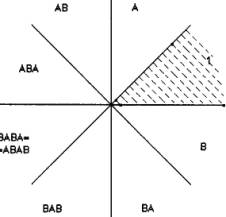Let G be the group generated by the reflections r and s in two lines r and s, making an angle of p/4 in point O. G is a finite group, consisting of four rotations (with center O and angles, respectively, p/2, p, 3p/2, 2p) and four reflections, with respect to the two lines r and s and other two lines t and u (see figure 1); the set of these four lines is the complete set of hyperplanes related to the group G (that is, the maximal set of hyperplanes such that the reflection with respect to that hyperplane belongs to the group); the connected components of the complement, in the plane, of these four lines are the chambers of the group G. All the chambers are equivalent (in the sense that there exists an element of the group sending one into the other), each one of them is an angle and the group is generated by the reflections in the walls of any chamber. There is a bijection between the whole set of the chambers and the elements of the group: fixing one of the chambers R0, the bijection is obtained by sending any other chamber R into the element g in G such that

g(R)= R0.

In order to consider another example, let H be the group generated by the reflections a, b, c in the three sides of a right isosceles triangle. H is an infinite group, and the complete set of hyperplanes is sketched in figure 2: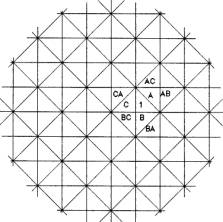It is an infinite grid, and the complement of this grid in the plane is made up of an infinity of isometric triangles, which are the chambers of the group.

It is worthwhile observing that, in both cases, the complete set of hyperplanes (and, as a consequence, also the set of the chambers) is just what we see if we start from a set of mirrors (two in the first case, three in the second one) corresponding to the generators of the group.

In fact, when we say that we are “seeing the group”, we are not referring only to the fact that the chambers we see are in one-to-one correspondence with the elements of the group, but, also, to the possibility of reading out from what we see the presentation of the group with generators and relations: in the case of figure 1, the group G is generated by r and s with relations r2 = s2 = (rs)4 = 1; in the case of figure 2, H has three generators a,b,c and relations a2 = b2 = c2 = (ab)2 = (bc)4 = (ac)4 = 1. In the photo, we can see another example, corresponding to thegfroup with three generators a, b, c and relations a2 = b2 = c2 = (ab)2 = (bc)3 = (ac)6 = 1.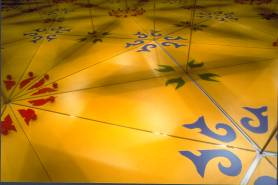This situation is quite general: any Coxeter group has a presentation with k generators g1,…,gk and relations of the kind (gigj)Nij=1; if i=j, Nii=1, as each gi is the reflection in a hyperplane; if i¹j, p/Nij is the angle between the two hyperplanes associated to the generators gi and gj.

From a geometric point of view, one can prove that any (irreducible)  Coxeter group is generated by the reflections on the walls of a chamber which can only be:

·     a simplex, if the group is infinite;

·     the cone on a simplex, if the group is finite.

There are other limitations on the shape of these chambers, which lead to the complete enumeration of Coxeter groups in any dimension; this is reached through an analysis which is quite lengthy in the general case, but, in low dimensions, is essentially a consequence of the observation that, due to the discreteness of the group, the dyhedral angles between the walls of the chamber must be of the form p/n, where n is any positive integral number (³2). This leaves very few possibilities, that is:

1.    for finite groups in two dimensions: two mirrors making an angle of p/n (for any n in N); the chamber is the cone on a 1-dimensional simplex;

2.    for infinite groups in two dimensions: three mirrors forming a triangle, with angles p/p, p/q, p/r. As 1/p + 1/q + 1/r = 1, the only possibilities are:

·     p=q=r=3: equilateral triangle;

·     p=2, q=r=4: right isosceles triangle;

·     p=2, q=3, r=6: right triangle with angles p/3 and p/6;

3.    for finite groups in three dimensions: three mirrors forming the cone on a triangle. The angles between the mirrors must be of the form p/p, p/q, p/r; as these are the angles of the corresponding spherical triangle, we get the inequality 1/p + 1/q + 1/r > 1, which leaves the only possibilities:

·     p=2, q=r=3: this corresponds to the symmetry group of a tetrahedron;

·     p=2, q=3, r=4: this corresponds to the symmetry group of a cube, or of a octahedron;

·     p=2, q=3, r=5: this corresponds to the symmetry group of a dodecahedron, or of an icosahedron.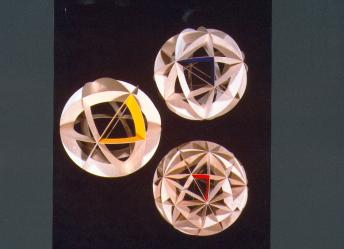The six cases just obtained in 2. and in 3. are exactly the six “machines for building symmetry” described in the introduction.

In order to complete the list of Coxeter groups acting in dimension less or equal to 3, we have to add also:

0.     groups in one dimension: there is only one finite group, corresponding to just one mirror; there is also only one infinite group, corresponding to two parallel mirrors.

4.    infinite groups in three dimensions: these are given by four mirrors forming a tetrahedron with all dyhedral angles of the form p/n; there are three possibilities, whose corresponding chambers are all shown in figure 3:

5.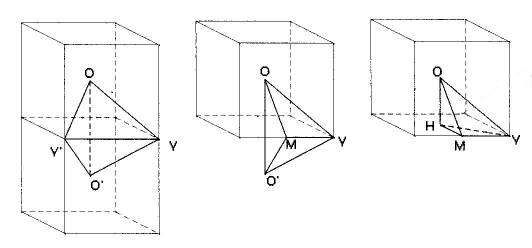·     the tetrahedron of vertices O,V,O’,V’, which has two dyhedral angles equal to p/2 and four equal to p/3;

·     the tetrahedron of vertices O,V,O’,M, which has three dyhedral angles equal to p/2, two equal to p/3 and one equal to p/4;

·     the tetrahedron of vertices O,V,H,M, which has three dyhedral angles equal to p/2, one equal to p/3 and two equal to p/4.

2.2        Two-dimensional machines and plane cristallographic groups

In the last subsection we found three irreducible cases among Coxeter infinite two-dimensional groups (equilateral triangle, right isosceles triangle, right triangle with angles p/3 and p/6) and one reducible case (rectangle). The same four cases appear among crystallographic groups, that is discrete groups of isometries in Rn, whose translation subgroup is generated by n independent translations. For n = 2, these are the 17 (up to affine conjugacy) wallpaper groups; among them, the ones which are generated by reflections are Coxeter groups (reducible or irreducible), and there are four of them:

·     p3m1 corresponding to the mirror box with the shape of an equilateral triangle;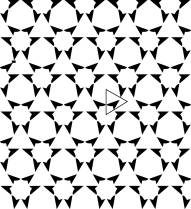·     p4m corresponding to the mirror box with the shape of a right isosceles triangle;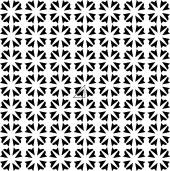·     p6m corresponding to the mirror box with the shape of a right triangle with angles p/3 and p/6;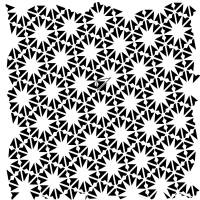·     pmm corresponding to the mirror box with the shape of a rectangle.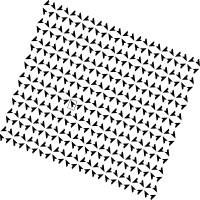It is also interesting to notice that these four groups are not the only ones – among wallpaper groups – which can be seen in our “machines”: in fact, when we put something in a mirror box, the planar picture we get has a symmetry group which contains the group G associated to the box (that is, the group generated by the reflections in the walls of the box), but not necessarily coincides with it. If we put in the box something which already has some symmetry, what we get is a group H which properly contains G as a subgroup and we may also “read” the index of G in H. So, if we want to make a list of which ones of the 17 wallpaper groups may be seen in our machines, we have to add some cases, that is:

·     p6m is not contained as a proper subgroup in any of the other 16 groups; so in the mirror box with the shape of a right triangle with angles p/3 and p/6 we can only see something with symmetry group p6m;

·     the same happens for the group p4m corresponding to the mirror box with the shape of a right isosceles triangle;

·     p3m1 is contained as a proper subgroup of (minimal) index 3 in the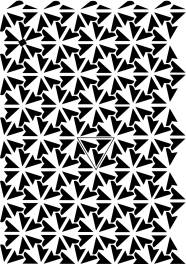crystallographic group p31m so, in the box with the shape of an equilateral triangle we generally see something with symmetry group p3m1 (by putting in the box something with no symmetry at all); but we can also see a planar picture with symmetry group p31m, by putting in the box something with symmetry group C3, that is a center of rotation of order 3 in the center of the mirror box. p3m1 is also contained as a proper subgroup of (minimal) index 2 in the crystallographic group p6m; this is for us less interesting, as it does not give a “new” group to see; however, in order to see the group p6m in the equilateral mirror box it is enough to put inside the box something with a bilateral symmetry.

·     pmm is contained as a proper subgroup

·     of (minimal) index 2 in the group cmm;

·     of (minimal) index 4 in the group p4g.

Besides these two cases, which add two new groups to the list of the ones we can see in mirror boxes, there are other two possibilities, as pmm is also contained as a proper subgroup

·     of (minimal) index 2 in the group p4m;

·     of (minimal) index 6 in the group p6m.

Thus in a rectangular mirror box we generally see something with symmetry group pmm (that is, this happens when we put inside the box something without any symmetry); we manage to get a picture with symmetry group cmm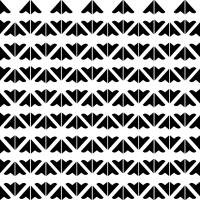when we put inside the box something with a center of symmetry of order 2 in the center of the rectangle. In order to get a picture with symmetry group p4g,we cannot start from any rectangular mirror box, but we need a square one,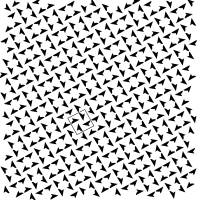and we have to put in the square box something with symmetry group C4, that is with a rotational center of order 4 in the center of the square. If we put in the square box something whose symmetry group is the dyhedral group D4 we get a picture with symmetry group p4m, but in fact we can get the same group (as a subgroup of index 2 instead of 8, that is by putting inside the box a picture with just a symmetry axis along the diagonal of the square. In order to get a picture with symmetry group p6m, we cannot start from any rectangular mirror box, but we need one such that the ratio between its sides is square root of 3 so that it can be divided in six right triangles with angles p/3 and p/6, each one obtained from the adjacent one by reflection in the common side: see figure 4.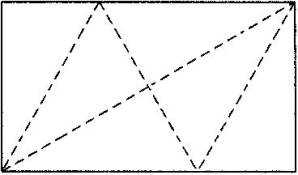So, the wallpaper groups which can be seen in a mirror box are seven, the four ones which are generated by reflections, and other three, which contain a subgroup generated by reflections.

2.3        Three-dimensional machines and polyhedra

And what about three-dimensional symmetry machines? The ones we described in the introduction realise the only possible irreducible finite subgroups of Iso(R3) generated by reflections, which correspond to the symmetry groups of the regular polyhedra. As in the two-dimensional case, the significative mathematical result hidden in these machines is the fact that they are not just an example, but they are in fact the only possible cases.

As in the two-dimensional case, by putting something in the “machine”, what one sees is the orbit F of that “something” with respect to the group associated to the caleidoscope (that is, the group generated by the reflections in the walls of the caleidoscope); the symmetry group of F contains the group of the caleidoscope, but does not necessarily coincides with it. However, finite groups of isometries in the space are very few, so that for two of the caleidoscopes (the one associated to the symmetry group of the cube and the one associated to the symmetry group of the dodecahedron) we may be sure that anything we see inside will always have a symmetry group which coincides with the group of the caleidoscope: the reason of this is simply the fact that there does not exist any finite group of space isometries containing as a subgroup either the symmetry group of the cube or the symmetry group of the dodecahedron.

Instead, in the caleidoscope associated to the symmetry group G of the tetrahedron  we may see either objects having G as symmetry group or objects having the same symmetry group H of a cube (as G is a subgroup of index 2 in H).

Colouring can be used (here as in the planar case) to underline this phenomenon. For example, an octahedron can be reproduced in the caleidoscope of the cube, but if we colour it with black and white faces (each black face touching only white ones, and viceversa) and we do want to reproduce that colouring also, then we need the caleidoscope of the tetrahedron.

Of course, regular polyhedra are not the only objects one can observe in the three-dimensional caleidoscopes. To give another example, we can consider the orbit F of a single point x with respect to the group associated to one of the three caleidoscopes, and the convex envelope of the points in F: in this way, we naturally get a uniform polyhedron (that is, a polyhedron whose symmetry group is transitive on the set of vertices). In particular, we get in this way nearly all the 13 Archimedean polyhedra:

·     in the caleidoscope of the tetrahedron  we get also the archimedean polyhedron (3,6,6);

·     in the caleidoscope of the cube we get the archimedean polyhedra (3,4,3,4), (4,6,6), (3,4,4,4), (3,8,8), (4,6,8);

·     in the caleidoscope of the dodecahedron we get the Archimedean polyhedra (3,5,3,5), (5,6,6), (3,4,5,4), (3,10,10), (4,6,10).

The only two archimedean polyhedra which can not be seen in the caleidoscopes are (3,3,3,3,4) and (3,3,3,3,5), whose symmetry groups contain only rotations.

The same construction does not only yield the Archimedean polyhedra (which, besides beeing uniform, have regular faces): in general, for any choice of x, we always get a polyhedron whose faces are equiangular; for each one of the described cases, there is just one position for the point x such that the faces of the corresponding polyhedron are also equilateral.

2.4        Elliptic, euclidean (and hyperbolic) geometry

Up to now we spoke about two-dimensional and three-dimensional machines for building symmetry; but there is another way - probably more suitable - to look at this situation. It should be noticed in fact that the world of polyhedra can be handled in (at least) two different ways: we can think to a polyhedron as a solid object (homeomorphic to D3), the analogous in the three-dimensional space of what is a polygon (homeomorphic to D2) in the plane; alternatively, we can think to a polyhedron as a two-dimensional object (homeomorphic to S2). From this point of view a polyedron is not so much the analogous of a plane polygon, but rather the analogous of a plane tessellation. With the first point of view one rather sees the symmetries of the object as isometries in the space; with the second point of view, one thinks more about isometries of the sphere.

This second point of view is probably the best one to underline the similarity between the different situations shown with our six “machines for building symmetry”. In any case we handle surfaces, more precisely triangulated surfaces; with the first three machines, the mirror boxes, we are in the world of euclidean geometry, and the restriction on the number of possible machines comes out essentially from the equality

1/p + 1/q + 1/r = 1,

implied by the fact that the sum of the angles of a euclidean triangle is equal to p; with the other three machines, the caleidoscopes, we are in the world of elliptic geometry, and the restriction on the number of possible machines comes out essentially from the inequality

1/p + 1/q + 1/r > 1,

implied by the fact that the sum of the angles of a spherical triangle is greater than p.

A very natural extension of this would be to have “machines for building symmetry” in hyperbolic geometry; and, in this case, we have a much greater variety, as there exists such a machine for any p, q, r such that

1/p + 1/q + 1/r < 1,

so there are an infinity of them. Each one corresponds to fixing a hyperbolic triangle whose angles are p/p, p/q, p/r and considering the subgroup G of hyperbolic isometries generated by the reflections in the sides of the triangle. There is no difficulty in simulating on a computer a virtual hyperbolic machine: it is enough (for example, in the Poincaré model) to substitute reflections with circular inversions. This is of course conceptually identical to a physical realization: however, in a plan for a math exhibition, a real object still makes a great difference, at least in my opinion, with respect to a virtual one (and it does not seem technically easy to construct such a machine).

3.    What can be done with the “machines for building symmetry”.

3.1        Classification with respect to symmetry, for different visitors

The principal aim of an exhibition based on the objects described in the introduction is to give the visitor an idea of the problem of classifying something with respect to its type of symmetry – an idea which of course will be at very different levels, depending on the degree of mathematical knowledge of the visitor.

The possibility of giving at least a flavour of this idea, even to someone with no mathematical knowledge at all (like small children) is due to the fact that, given a group generated by reflections, it is possible to express the idea of isomorphic or non-isomorphic groups without any technical algebraic language, but simply by looking at the geometry of the mirrors.

This opens the possibility of making a lot of non trivial considerations, related to the symmetry group of a planar picture or of a solid object, without the necessity of introducing the algebraic language related to groups; for example, planar pictures which can be reconstructed in the same mirror box (or solid objects which can be reconstructed in the same caleidoscope) have isomorphic symmetry groups, while pictures (or solid objects) reconstructed in different mirror boxes (or caleidoscopes) have non-isomorphic symmetry groups.

Of course, due to what we observed in the preceding section, the last sentence is in fact not quite correct. However, we do not think this ambiguity makes a serious problem towards mathematical communication in such a sort of exhibition. In fact, for the minority of the public who can appreciate this difference, the ambiguity is not hidden but, on the contrary, some problems are posed on purpose, in order to provoke questions on the subject, and this gives a new, not-trivial problem to investigate. For the majority of the public, in order to give a flavour of the different types of symmetry, it is enough to make them observe how the pictures coming out from certain mirror boxes are all based on numbers 3 or 6 (and on a triangular grid), while others are based on number 4 (and on a square grid).

Moreover, the same problems can be used for the more and for the less sophisticated public with different purposes. To give just one example, the reconstruction of the same tessellation with different kinds of colouring (which can also lead to different symmetry groups) can be used with the more sophisticated public precisely to provoke the ambiguity we were discussing before, while with the less sophisticated one the same problem can be used for simpler observations.

In fact, one of the reasons why we thought the “machines for building symmetry” were useful for an exhibition is exactly what is exemplified in the previous comment, that is the fact that the same objects can be used to communicate different levels of mathematics to different sorts of public. The fact that they are based on some non-trivial mathematical concepts from one side allows also an interesting communication towards a public with more mathematical background and from another side it is clearly perceivable also by the public with very little mathematical knowledge: often, it is not necessary to be able to enter deeply inside a problem in order to understand whether the problem has, or has not, such a depth.

3.2        The role of interactivity

Another reason why we think these machines can be a useful example in the direction of finding ways for the popularization of mathematics is the fact that they give occasions of “doing mathematics”; and, in saying this, we think both to the public with less mathematical knowledge and to the public with more technical instruments. Both will have the possibility of putting their hands on the objects and meet a problem they will have to solve: for a seven-year old child the problem can be how to put a given triangle in a mirror box in order to see an hexagon; for a mathematics university student the problem can be that of understanding why a crystallographic group cannot have order 5 rotations, … : in both cases (as well as with other possible categories of public) the objects can provoke an active reaction by the visitor: which we think is the only effective way to learn some mathematics.

Another aspect, related to this one, is the crucial role played by fancy and creativity, which are human capacities usually (and wrongly) thought to be far away from mathematical capacities. This crucial role comes out from the fact that the main thing to do with our machines is just to look what happens when one puts something inside, and to observe analogies and differences between them.

In order to make these observations, any object would do: if I take the piece which represents the  fundamental domain of the action of the symmetry group of the cube and I put it, in the “right” way, in the caleidoscope of the cube, I see a cube.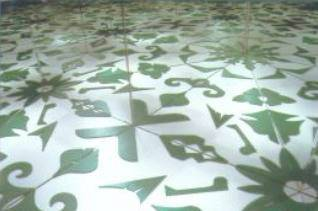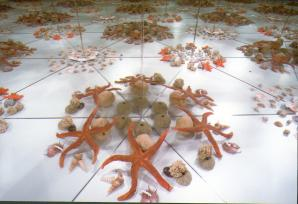But if I put it in a “wrong” way, or I do not put that piece at all, but I prefer to insert a ball, or even a dry flower I had in my pocket, that will work equally well; in this case also I can observe that what I see has always the same kind of symmetry of a cube and always a kind of symmetry different from what we see in the other caleidoscopes.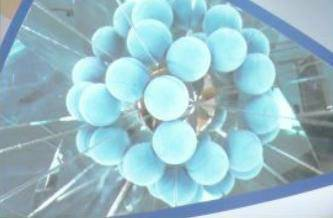So, the “wrong” trials to solve the problems proposed are equally well useful to observe what happens and thus familiarize with the concepts involved; this may be very “relaxing”, especially for people (unfortunately not so rare) who are paralysed by a sort of “fear” towards maths.

3.3        The role of mathematical proofs

We spoke of active interaction with the objects, and of fancy, and of playing. But mathematics is also (or mainly?) rigorous proofs. What could the role of proof be in this kind of proposal? In fact, the problem of achieving a rigorous proof is (always, but especially the context of undergraduate teaching) a matter of subsequent approximations. And the first stages of these approximations are the understanding of what has to be proved, and the consciousness that the given fact is not trivial and has thus to be proved. This seems (and in fact is) an obvious consideration, but it is unfortunately frequently forgotten: pupils are often forced to prove a statement in the moment when they have not yet clear ideas about what it does mean (what it means if it happens to be true, what would happen if it is false,… ecc.); or they may be asked to prove some facts which are eventually not so easy to prove, but whose statement is (or appears) evident.

It is of course one of the main aspects of mathematics the fact that, in a deductive construction, one has to prove everything, also “self-evident” statements, and we perfectly know how some self-evident statements are not at all trivial to prove (Jordan’s theorem, just to give an example), and some are even false. But …; but one needs some mathematical maturity to appreciate the need to prove self-evident statements; and it can be useless, or even damaging, to propose their proof in a context where this maturity is lacking.

This brings to the (apparent) paradox that it may be easier to propose the proof of “difficult” statements than that of “easy” ones in secondary school. The role of a mathematical exhibition towards the achieving of proofs could well be (on some categories of public) that of making people conscious, and curious, about some facts to be proved.

Let us exemplify what we are trying to say on two statements: the first one is the fact that a triangle with two equal sides has two equal angles; the second one is the fact that the frieze groups are seven. And let us keep in mind two sorts of public, a secondary school student and an adult with no mathematical background after school. It is very unlikely that both the student or the grown-up person manage to become particularly curious about the statement on isosceles triangles; in any case they both believe it is true, and they would use the statement, without realising it requires a proof, if in a concrete problem they happen to need it. The situation is completely different for the statement about friezes: first of all the statement is strange, and difficult to understand; one does not understand it at once, but has to think about it, to make a list of the possibilities, to reason about the fact that whatever drawing he or she is making, it has to be one of those seven. When one grasps what this means, usually this is related to a sense of beauty: the result is beautiful, conceptually beautiful. Moreover, it looks strange; and it is very natural to ask why is an eighth case impossible. So, with some time at disposal, it is very easy that people arrive naturally to the consciousness of the need for a proof.

When we have obtained such a consciousness, there are still many intermediate stages which can be significant, before achieving a complete proof: for example, one could begin to observe that, due to the fact that the group is a frieze group, there must be some restrictions on the kind of possible isometries in the group (rotations may only be of order two; the axis of reflections may only be either parallel or perpendicular to the direction of translations; the axis of glide reflections must be parallel to the direction of translations; …). This is not yet a proof, but it begins to give some flavour of it, and the statement, which at first sight appeared completely mysterious (“why just seven???”) can now be seen as more reasonable (“I still do not know why they are exactly seven, but I do understand that the situation is not completely free, and there are some limitations to be respected”).

In fact, these intermediate stages may be exactly what we would like to be grasped about proofs in mathematics undergraduate learning, much more than the particular proof of a particular statement, which is often not relevant in itself (at least at that level).

3.4        The role of beauty

Beauty has – in many different ways – a crucial role in the exhibition just described.

The first aspect regards a problem of motivations. We all know very well that mathematics has on the whole a very bad reputation; it is quite common to meet persons who have a sort of hate and/or fear towards mathematics; there may be also (in the same person) interest or curiosity about maths, besides fear, but it is very likely that fear acts as a sort of block towards curiosity. This block is a concrete problem that any trial of popularizing maths has to consider; one needs a way to overcome it, to be able to begin to communicate with the public; and, moreover, this way must be an immediate one, because it has to win an irrational feeling, not a rational one.

The strong impact of beauty is an enormous help in this sense; and we found symmetry a very good subject for popularization of maths also for this reason (besides the ones already discussed).

By saying this, we do not only refer to the trial of involving art, by proposing posters with the reproductions of some masterpieces where symmetry is wonderfully used; but we refer also to the “beauty” of the artificial images that the visitor is invited to reconstruct (and/or to invent) by himself. Of course these two kinds of “beauty” are not comparable, but it is a fact that both have a precious role in disposing people to be willing to interact with maths – a result which is not at all obvious to reach.

A particular role is played by the strong effect of surprise. In our mirror boxes one “can see infinity” and this effect is very strong, very beautiful, and also very unexpected, in all sort of public.

Moreover, this effect of surprise does not come from spectacular, enormous, scenic objects, but from very simple ones. This has a double positive effect: the first one is that it magnifies the surprise (if I have to enter into an enormous building, which I see from very far away, with much light and colour, I do expect I shall see something which will surprise me; maybe I have no idea of what I shall see, so I shall still be surprised, but I know in advance that this will happen; instead, if I put my eye on the border of an object which looks very simple, I do not expect any particular “special effect”); another positive consequence is that the objects are easily reconstructible, so for example teachers realize they can easily build something analogous in their schools.

A last aspect about beauty I would like to remind here is one which has already been mentioned in the preceding section: mathematics is beautiful not only for the beauty of some of its images, but, also, for the conceptual beauty of some of its results. Too often – in my opinion – we do not even try to communicate this kind of beauty: mathematicians seem to lack any confidence about the fact that this could be communicated to someone, unless he or she has done the right number of exams in algebra, geometry, analysis ecc. Sometimes this is true, but it is probably much less true than what is generally thought, and it is possible to communicate much more than we think. At least, it is worthwhile trying.

 D is a fundamental domain for the action of G on P if for any point x in P we can find an element g in G such that g(x) belongs to D and no two points in the interior of D are related by an element in G.

 This is in fact just a first rough approximation, which is not quite correct: see the following section for a more precise statement.

 A Coxeter group is irreducible if it is not isomorphic to the direct product of two Coxeter groups acting in lower dimensions.

 If we try to construct a plane polygon with all angles of the form p/n, there is another possibility besides these three triangles, that is a rectangle, with all angles p/2. This case is less significative among Coxeter groups, because it is a reducible group: in fact, it can be seen as the direct product of two copies of the group generated by the reflections in two parallel lines, each copy acting over R.

 The index of G in H is equal to the ratio of the areas of the fundamental domains of G and H:  this can easily be read from what we see, keeping in mind that the fundamental domain of G is the box itself.

 As p6m is a subgroup of itself, what can happen is that by putting something in the box we may get a picture whose symmetry group H is always isomorphic to p6m, BUT is different from the group G generated by the reflections in the walls of the box.

 As p4m is a subgroup of itself, the same phenomenon described in note 6 for  p6m can happen.

 In fact, the symmetry group of the octahedron is isomorphic to H, while the coloured symmetry group (that is, the subgroup of those isometries which, besides fixing the object, fix that colouring also) is isomorphic to G.

 The notation (a1,a2,…ak) used for an archimedean polyhedron expresses the fact that each vertex is adjacent to a regular a1-gone, a regular a2-gone …, a regular ak-gone (in this cyclical order).

 The distinction between two- and three-dimensional situation can generate ambiguity in a context where the objects are phisycally shown (and, therefore, are all necessarily three-dimensional).

 For example, we can build in a square mirror box pictures having four possible (non isomorphic) symmetry groups (pmm, cmm, p4m, p4g); while a picture with symmetry group p4m could be built both in a square mirror box or in a mirror box with the shape of a right isosceles triangle.

 Frieze groups are the symmetry groups of patterns repeating in just one direction; that is, they are discrete subgroups of the group of plane isometries, whose translation subgroup is isomorphic to Z.

 Although this is on the whole a very positive fact, it also poses some  problems: in fact, it is not always easy tokeep control on this and to prevent imitations by people who are misunderstanding the conceptual and didactical role of the objects.

BIBLIOGRAPHY

 H.S.M. COXETER, Introduction to geometry, Wiley (1961)

 H.S.M. COXETER, J. MOSER, Generators and relations for discrete groups, Springer (1980)

 P. CROMWELL, Polyhedra, Cambridge University Press (1997 )

 M. DEDÒ,  Forme, Decibel-Zanichelli (1999)

 H. WEYL, Symmetry, Princeton University Press (1952)

Dipartimento di Matematica “F. Enriques” Università di Milano

 All the photos are taken from the exhibition “Simmetria, giochi di specchi” and made by Sabrina Provenzi ; reproduction authorized by the head of the Maths Department “F. Enriques” of Milan University.